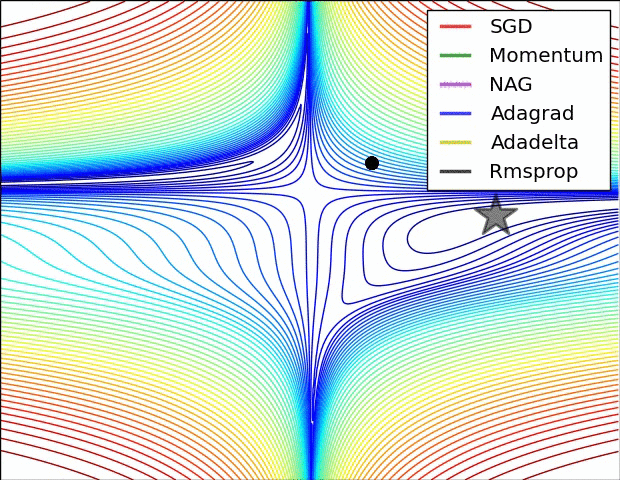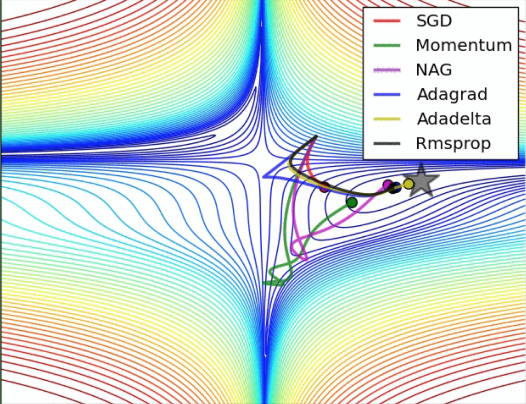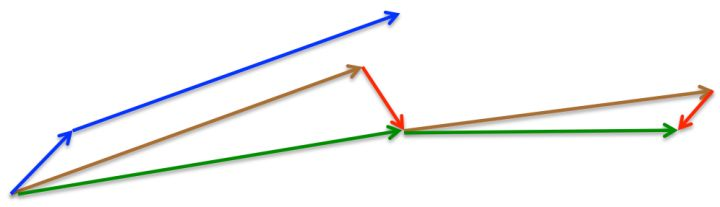2018-07-04 21:19:17 fengchi863 阅读数 438
• ###### 玩转深度学习实战教程

玩转深度学习视频培训课程，详细讲解深度学习的原理和利用深度学习框架TensorFlow进行项目实战。课程通过Kaggle竞赛平台的Titanic问题讲解TensorFlow的基本用法以及问题处理的常用技巧，讲解深度学习图像领域的卷积神经网络CNN和多个经典的网络架构、CNN的应用，讲解自然语言处理领域的RNN、LSTM以及它们的多种变种结构，构建语言模型和对话机器人，介绍损失函数和优化深度学习算法在TensorFlow中的实现。

3209 人正在学习 去看看 王而川

1. 对于大规模的神经网络，大多数的局部最优都有一个比较低的损失值，并且寻找真实的全局最优也不是一个很重要的问题，重要的是在参数空间中找到一个相对较低的局部最优值。（因为全局最优可能过拟合严重，局部最优反而可能更好）
2. 鞍点：是高维数据中一个很突出的问题。鞍点就像是两座山峰的中间区域，该区域不是局部最优值，但是十分平坦，换言之就是梯度几乎为0，无法确定走哪一条路。维度越高，鞍点的数目越是指数型增长。
3. 梯度悬崖：由于循环神经网络涉及在多个时间段内相乘，所以梯度悬崖在RNN中十分频繁，特别是处理较长的时间序列时。
4. 梯度消失与梯度爆炸：比如Sigmoid神经元，取值范围为(0,0.25]，在梯度反向传播时，当层数较多时，最终导数值已经很小，所以即使网络产生了很大的误差，但底层的神经元依然没有得到足够的误差修正。反之，如果上层的权重过大，当经过传递后，本层的梯度就会变得异常巨大，造成梯度十分不稳定。相比于梯度消失，梯度爆炸的问题比较容易解决，并且发生的情况也不频繁。
5. 随机梯度下降（SGD）：随机梯度下降算法就是选择一条数据，就训练一条数据，然后修改一次权重。为了防止这种随机性带来的危害，我们就多选择几条数据，计算一下多条数据的平均错误，如果某条数据存在严重错误，也会因为多条数据的中和而降低其错误的成都。
6. 为了防止在最优解附近震荡的现象，我们可以把学习率改为时间衰减的形式。如下式，为训练第$k$$k$轮时第$i$$i$轮学习率的取值。
${\alpha }_{i}=\left(1-\frac{i}{k}\right){\alpha }_{0}+\frac{i}{k}b$

当使用这种线性策略来调整学习率时，通常要进行上百轮的学习调整，因此$k$$k$要大于100，$b$$b$的取值可以粗略的设置为百分之一的学习率。设置初始学习率很重要，如果太大，学习曲线就会剧烈震荡；如果太小，学习就会非常慢，还可能会陷入一个比较高的代价函数区域。这也是一个鱼和熊掌的问题。
7. 动量学习法：梯度的物理意义可以理解为，是一个力，带领我们下山，但是我们不是靠力前行，而是靠速度，力只是改变速度的大小和方向，并且速度是可以累积的，所以我们还具有动量。类似于物理学，速度这个参数表示在参数空间移动的方向和速率，而代价函数的负梯度表示参数在参数空间移动的力。引入超参数$\beta$$\beta$，它的值在[0,1]范围之间，用于调节先前梯度（力）的衰减效果。
$v=\beta v-\alpha \mathrm{\Delta }w$

$w=w+v$

对上式进行变换，可以得到
${v}_{max}=\frac{\alpha ||w||}{1-\beta }$

在动量学习法中，每一步走多远依赖的是过去的速度以及当前的力（梯度）。速度$v$$v$用于累加各轮训练的参数梯度（类似有个加速度），$\beta$$\beta$越大先前梯度对于本轮训练的影响就越大。最终因为衰减因子$\beta$$\beta$的存在，小球在达到最大值以后就会匀速前行。（理解为一个受力平衡的状态）常用的$\beta$$\beta$可以是0.5,0.9.0.99。
对于$\beta$$\beta$的调整，并没有$\alpha$$\alpha$的调整中重要，所以不太需要作为超参数进行选择，正常情况下，取值适当即可。
$cach{e}_{i}=cach{e}_{i}+\left(\mathrm{\Delta }{w}_{i}{\right)}^{2}$

${w}_{i}={w}_{i}-\frac{\alpha }{\sqrt{cach{e}_{i}}+\delta }\mathrm{\Delta }{w}_{i}$
9. RMSProp：因为AdaGrad在理论上有比较好的性质，但是对优化神经网络十分不友好，随着训练周期的增长，学习率降低的很快。RMSProp算法在进行梯度累积的时候，会在“过去”和“现在”之间做一个权衡，通过超参数$\beta$$\beta$来条件衰减量，常用取值0.9或0.5。
$cach{e}_{i}=\beta cach{e}_{i}+\left(1-\beta \right)\left(\mathrm{\Delta }{w}_{i}{\right)}^{2}$

${w}_{i}={w}_{i}-\frac{\alpha }{\sqrt{cach{e}_{i}}+\delta }\mathrm{\Delta }{w}_{i}$

RMSProp更新方式对于深度学习网络十分的高效，是深度学习中最有效的更新方式之一。
$g=\frac{1}{m}\sum _{j=1}^{m}\frac{\mathrm{\partial }L\left({y}^{\left(i\right)},f\left({x}^{\left(j\right)};w\right)\right)}{\mathrm{\partial }w}$

类似于动量学习法，设置衰减梯度$v$$v$:
$v={\beta }_{1}v+\left(1-{\beta }_{1}\right)g$

类似于RMSProp学习法，设置衰减学习率$r$$r$:
$r={\beta }_{2}r+\left(1-{\beta }_{2}\right){g}^{2}$

最后更新参数：
$w=w-\frac{\alpha }{\sqrt{r}+\delta }v$

因为在开始的时候梯度会非常的小，$r$$r$$v$$v$经常会接近于0，所以做下面的“热身”工作：
$vb=\frac{v}{1-{\beta }_{1}^{t}},rb=\frac{r}{1-{\beta }_{2}^{t}}$

其中，$t$$t$表示训练的次数，因此仅仅在训练的前几轮中根据就衰减因子来放大各自的值，很快$vb$$vb$$rb$$rb$就会退化成$v$$v$$r$$r$
11. 参数初始化策略：无论使用何种学习方式，都避免不了同一个问题，那就是下山的起始点问题，也就是参数的初始化问题。初始化不仅印象训练效果，还能影响模型的泛化性能（输在起跑线上）。
12. 应该尽量避免神经元参数出现对称的情况。如果两个神经元使用相同的激活函数，并且输入相同，那么每个神经元都要有不同的参数。否则因为参数相同，在确定的学习算法下，他们的参数的更新也就会相同，出现神经元冗余的情况。
13. 一般采用均匀分布或者高斯分布的随机初始化方式，分布函数的取值范围（标准差）对优化过程以及泛化能力有着巨大的影响。
较大的初始化权重范围可以有效避免参数对称线性，减少神经元冗余现象，还可以帮助减轻训练网络时的梯度消失问题，但是太大的初始化权重也会导致梯度爆炸的问题，在一些Sigmoid神经元中，也容易出现过饱和现象，导致修改神经元梯度几乎为0。
14. 一般参数均值要为0，这是一条高斯分布先验知识。通常，在$m$$m$输入$n$$n$输出的单层神经元中，我们可以使用均匀分布对权重进行随机采样初始化。
${W}_{i,j}\sim U\left(-\frac{1}{\sqrt{m}},\frac{1}{\sqrt{m}}\right)$

或者使用标准初始化：
${W}_{i,j}\sim U\left(-\frac{6}{\sqrt{m+n}},\frac{6}{\sqrt{m+n}}\right)$
15. 批量归一化：BN层，不能算是一种最优化算法，但是很有用。与选择最小批量梯度下降一样，BN中的“批量”就是采样一小批数据，然后对该批数据在网络各层的输出进行归一化处理。BN的目的就是为了把神经网络每一层的输入数据都调整到均值为零，方差为1的标准正态分布
Sigmoid在[-2,2]之间的取值是一段近似线性的区域，BN算法所做的就是把输入值尽可能的归一化在激活函数这一狭窄区域。
BN算法还有另一个步骤，就是将归一化的数据放大，平移回非线性区域。

2019-06-15 21:45:23 x454045816 阅读数 122
• ###### 玩转深度学习实战教程

玩转深度学习视频培训课程，详细讲解深度学习的原理和利用深度学习框架TensorFlow进行项目实战。课程通过Kaggle竞赛平台的Titanic问题讲解TensorFlow的基本用法以及问题处理的常用技巧，讲解深度学习图像领域的卷积神经网络CNN和多个经典的网络架构、CNN的应用，讲解自然语言处理领域的RNN、LSTM以及它们的多种变种结构，构建语言模型和对话机器人，介绍损失函数和优化深度学习算法在TensorFlow中的实现。

3209 人正在学习 去看看 王而川

• Momentum optimization
• RMSProp

### 1. 回顾

a. 选择一个合适的权重初始化策略
b. 用一个合适的激活函数
c. 运用Batch Normalization策略
d. 复用之前的网络模型

### 2. Momentum optimization

#### 2.1 原理

momentum optimization可以翻译为动量优化器，该优化器背后的思想很简单: 假设一个圆球从一个斜坡上滚下去，一开始速度会很慢，但是随着时间的增加，速度会越来越快，考虑到摩擦力和空气阻力，最终会达到一个恒定的速度（空气阻力跟速度的平方成正比）。这就是momentum optimization 背后的思想。跟一般的梯度下降法相比，显然动量优化速度更快。

$\theta\leftarrow\theta-\eta{\nabla_\theta}J(\theta)$

$1.\quad m \leftarrow \beta m+ \eta{\nabla_\theta}J(\theta)$
$2.\quad \theta \leftarrow \theta - m$

#### 2.2 实现

optimizer = tf.train.MomentumOptimizer(learning_rate=learning_rate,
momentum=0.9)


#### 3.1 原理

Nesterov Accelerated Gradient（NAG）算法，其实是对Momentum Optimization的一个小小的改进，在动力优化中，更新动力变量$m$是在当前($\theta$)的梯度上进行更新的，而在NAG算法中，对动力变量的更新在动力方向上稍微提前了一些($\theta + \beta m$)，如下:

$1.\quad m \leftarrow \beta m+ \eta{\nabla_\theta}J(\theta + \beta m)$
$2.\quad \theta \leftarrow \theta - m$

#### 3.2 实现

optimizer = tf.train.MomentumOptimizer(learning_rate=learning_rate,
momentum=0.9, use_nesterov=True)


#### 4.1 原理

$1.\quad s \leftarrow s + {\nabla_\theta}J(\theta) \otimes {\nabla_\theta}J(\theta)$
$2.\quad \theta \leftarrow \theta - \eta {\nabla_\theta}J(\theta) \oslash {\sqrt{s+\varepsilon}}$### 5. RMSProp

#### 5.1 原理

$1.\quad s \leftarrow \beta s + (1 - \beta){\nabla_\theta}J(\theta) \otimes {\nabla_\theta}J(\theta)$
$2.\quad \theta \leftarrow \theta - \eta {\nabla_\theta}J(\theta) \oslash \sqrt{s+\varepsilon}$

#### 5.2 实现

optimizer = tf.train.RMSPropOptimizer(learning_rate=learning_rate,
momentum=0.9, decay=0.9, epsilon=1e-10)


#### 6.1 原理

$1.\quad m \leftarrow \beta_1 m+ (1 - \beta_1){\nabla_\theta}J(\theta)$
$2.\quad s \leftarrow \beta_2 s + (1 - \beta_2){\nabla_\theta}J(\theta) \otimes {\nabla_\theta}J(\theta)$
$3.\quad m \leftarrow \frac {\bm m} {1 - {\beta_1}^T}$
$4.\quad s \leftarrow \frac {\bm s} {1 - {\beta_2}^T}$
$5.\quad \theta \leftarrow \theta - \eta m \oslash \sqrt{s+\varepsilon}$

#### 6.2 实现

optimizer = tf.train.AdamOptimizer(learning_rate=learning_rate)


### 7. 小结

（如需更好的了解相关知识，欢迎加入智能算法社区，在“智能算法”公众号发送“社区”，即可加入算法微信群和QQ群）2017-09-14 15:32:20 chanbupt 阅读数 854
• ###### 玩转深度学习实战教程

玩转深度学习视频培训课程，详细讲解深度学习的原理和利用深度学习框架TensorFlow进行项目实战。课程通过Kaggle竞赛平台的Titanic问题讲解TensorFlow的基本用法以及问题处理的常用技巧，讲解深度学习图像领域的卷积神经网络CNN和多个经典的网络架构、CNN的应用，讲解自然语言处理领域的RNN、LSTM以及它们的多种变种结构，构建语言模型和对话机器人，介绍损失函数和优化深度学习算法在TensorFlow中的实现。

3209 人正在学习 去看看 王而川

http://cs231n.github.io/neural-networks-3/#sgd
·······················································································································

# 随机梯度下降方法及其变种

## Vanilla update

x += -learning_rate * dx
Momentum update

v = mu * v – learning_rate *dx # 整合后的速度
x += v # 整合后的位置

“With Momentum update, the parameter vector will buildup velocity in any direction that has consistent gradient.（随着动量的更新，参数向量将会建立起在所有方向上都恒定梯度的速度）”

## Nesterov Momentum

Nesterov动量是与动量更新略微不同的一个版本，最近变得流行起来。它对凸函数的收敛有着很强的理论保证，并且实践中也比标准的动量更新方式效果稍微更好些。
Nesterov动量更新的关键思想是，当目前的参数在某个位置x时，我们知道单独的动量项（即忽略具有梯度的第二项）即将推动参数 矢量由mu * v。因此，我们要计算梯度时，我们可以将未来的近似位置x + mu * v视为“前瞻”（lookahead） - 这是我们即将到达的地方附近。因此，计算在x + mu * v处的梯度比“旧”的位置x上的梯度更有意义。X_head = x + mu * v
v = mu * v – learning_rate *dx_ahead
x += v

# 参数自适应学习方法

   我们迄今为止所讨论的所有以前的方法在全局和所有参数中均衡地学习了学习率。调整学习率是一个昂贵的过程，因此设计可以自适应地调整学习率的方法，甚至每个参数都做了很多工作。 这些方法中的许多可能仍然需要其他超参数设置，但是论点是相比原始学习速率，他们对于范围更广泛的超参数值表现更好。


cache+= dx**2
x +=-learning_rate * dx / (np.sqrt(cache) + eps)

## RMSporp

RMSprop是一种非常有效的，但目前尚未发布的自适应学习率方法。 RMSProp更新以非常简单的方式调整Adagrad方法，以减少其激进，单调降低的学习率。特别地，它使用平均渐变的移动平均值，给出：

cache = decay_rate * cache +(1 – decay_rate) * dx**2
x += -learning_rate * dx /(np.sqrt(cache) + eps)

Adam是最近提出的，看起来像RMSProp的一种基于动量的方法。 （简化版）更新方法如下所示：

m = beta1 * m + （1-beta1）* dx # 动量
v = beta2 * v + (1-beta2) *(dx**2) # cache
x += -learning_rate * m /(np.sqrt(v) + eqs)

m = beta1*m + (1-beta1)*dx
mt = m / (1-beta1**t)
v = beta2*v +(1-beta2)*(dx**2)
vt = v / (1-beta2**t)
x += - learning_rate * mt /(np.sqrt(vt) + eps)

# 各种参数更新方法的可视化2018-09-19 20:19:17 SoundSlow 阅读数 2111
• ###### 玩转深度学习实战教程

玩转深度学习视频培训课程，详细讲解深度学习的原理和利用深度学习框架TensorFlow进行项目实战。课程通过Kaggle竞赛平台的Titanic问题讲解TensorFlow的基本用法以及问题处理的常用技巧，讲解深度学习图像领域的卷积神经网络CNN和多个经典的网络架构、CNN的应用，讲解自然语言处理领域的RNN、LSTM以及它们的多种变种结构，构建语言模型和对话机器人，介绍损失函数和优化深度学习算法在TensorFlow中的实现。

3209 人正在学习 去看看 王而川• Optimizer 主类：

• MomentumOptimizer
Momentum单词即动量、动力，这里的意思可以理解为惯性，想象一下，如果你现在从山上玩下冲，你的目的是到达最低点，但是如果这个山坡并不是直线梯度下降，而是局部左拐右拐梯度下降，这样将导致下降步骤是个折线形，大大降低训练速度，这也是基本梯度下降算法的缺点，因此这里引入了动量因子。
所以你下山有之前的动量，即便你想拐弯都拐不了很大
现在变为：variable -= learning_rate * (momentum * accumulation + gradient)
这里的momentum * accumulation是之前的动量积累，所以就导致即便局部有折线，也会由于之前的动量影响太大，而尽量保持直线下降
源码公式：
accumulation = momentum * accumulation + gradient
variable -= learning_rate * accumulation
或者直接是：W += b1 * m -Learning rate * dx
公式变化如下：
W += -Learning rate * dx
变为：
v += dx^2
W += -Learining rate * dx /sqrt(v)
同增加惯性因子的原理类似，此方法也是为了减轻局部偏折，但是作用在学习率上。即为了防止偏折过大，我们会首先计算偏折幅度-即梯度值，并将其作为学习率分母参数。意思就是，如果梯度很大，学习率就很低，这样防止偏折过大；梯度很小，学习率就大。
• RMSPropOptimizer
公式：
V = b2* V +(1-b2) * dx^2
W += -Learining rate * dx /sqrt(v)

公式：
M += b1 * m -Learning rate * dx (momentum)
W += -Learining rate * M /sqrt(V)

2018-03-15 17:24:29 u013498583 阅读数 287
• ###### 玩转深度学习实战教程

玩转深度学习视频培训课程，详细讲解深度学习的原理和利用深度学习框架TensorFlow进行项目实战。课程通过Kaggle竞赛平台的Titanic问题讲解TensorFlow的基本用法以及问题处理的常用技巧，讲解深度学习图像领域的卷积神经网络CNN和多个经典的网络架构、CNN的应用，讲解自然语言处理领域的RNN、LSTM以及它们的多种变种结构，构建语言模型和对话机器人，介绍损失函数和优化深度学习算法在TensorFlow中的实现。

3209 人正在学习 去看看 王而川

SGD

SGD就是每一次迭代计算mini-batch的梯度，然后对参数进行更新，是最常见的优化方法了。即：

${g}_{t}={\mathrm{\nabla }}_{{\theta }_{t-1}}f\left({\theta }_{t-1}\right)$

$\mathrm{\Delta }{\theta }_{t}=-\eta \ast {g}_{t}$

SGD完全依赖于当前batch的梯度，所以\eta可理解为允许当前batch的梯度多大程度影响参数更新

- 对所有的参数更新使用同样的learning rate。对于稀疏数据或者特征，有时我们可能想更新快一些对于不经常出现的特征，对于常出现的特征更新慢一些，这时候SGD就不太能满足要求了

SGD容易收敛到局部最优，并且在某些情况下可能被困在鞍点【原来写的是“容易困于鞍点”，经查阅论文发现，其实在合适的初始化和step size的情况下，鞍点的影响并没这么大。感谢@冰橙的指正】

Momentum
momentum是模拟物理里动量的概念，积累之前的动量来替代真正的梯度。公式如下：

${m}_{t}=\mu \ast {m}_{t-1}+{g}_{t}$

$\mathrm{\Delta }{\theta }_{t}=-\eta \ast {m}_{t}$

Nesterov
nesterov项在梯度更新时做一个校正，避免前进太快，同时提高灵敏度。

$\mathrm{\Delta }{\theta }_{t}=-\eta \ast \mu \ast {m}_{t-1}-\eta \ast {g}_{t}$

${g}_{t}={\mathrm{\nabla }}_{{\theta }_{t-1}}f\left({\theta }_{t-1}-\eta \ast \mu \ast {m}_{t-1}\right)$

${m}_{t}=\mu \ast {m}_{t-1}+{g}_{t}$

$\mathrm{\Delta }{\theta }_{t}=-\eta \ast {m}_{t}$momentum首先计算一个梯度(短的蓝色向量)，然后在加速更新梯度的方向进行一个大的跳跃(长的蓝色向量)，nesterov项首先在之前加速的梯度方向进行一个大的跳跃(棕色向量)，计算梯度然后进行校正(绿色梯向量)

${n}_{t}={n}_{t-1}+{g}_{t}^{2}$

$\mathrm{\Delta }{\theta }_{t}=-\frac{\eta }{\sqrt{{n}_{t}+ϵ}}\ast {g}_{t}$

$\eta$$\eta$设置过大的话，会使$regularizer$$regularizer$过于敏感，对梯度的调节太大

${n}_{t}=\nu \ast {n}_{t-1}+\left(1-\nu \right)\ast {g}_{t}^{2}$

$\mathrm{\Delta }{\theta }_{t}=-\frac{\eta }{\sqrt{{n}_{t}+ϵ}}\ast {g}_{t}$

$E|{g}^{2}{|}_{t}=\rho \ast E|{g}^{2}{|}_{t-1}+\left(1-\rho \right)\ast {g}_{t}^{2}$

$\mathrm{\Delta }{x}_{t}=-\frac{\sqrt{\sum _{r=1}^{t-1}\mathrm{\Delta }{x}_{r}}}{\sqrt{E|{g}^{2}{|}_{t}+ϵ}}$

RMSprop

$\rho =0.5$$\rho=0.5$时，$E|{g}^{2}{|}_{t}=\rho \ast E|{g}^{2}{|}_{t-1}+\left(1-\rho \right)\ast {g}_{t}^{2}$$E|g^2|_t=\rho*E|g^2|_{t-1}+(1-\rho)*g_t^2$就变为了求梯度平方和的平均数。

$RMS|g{|}_{t}=\sqrt{E|{g}^{2}{|}_{t}+ϵ}$$RMS|g|_t=\sqrt{E|g^2|_t+\epsilon}$

$\mathrm{\Delta }{x}_{t}=-\frac{\eta }{RMS|g{|}_{t}}\ast {g}_{t}$$\Delta{x_t}=-\frac{\eta}{RMS|g|_t}*g_t$

- 对于RNN效果很好

${m}_{t}=\mu \ast {m}_{t-1}+\left(1-\mu \right)\ast {g}_{t}$

${n}_{t}=\nu \ast {n}_{t-1}+\left(1-\nu \right)\ast {g}_{t}^{2}$

$\stackrel{^}{{m}_{t}}=\frac{{m}_{t}}{1-{\mu }^{t}}$

$\stackrel{^}{{n}_{t}}=\frac{{n}_{t}}{1-{\nu }^{t}}$

$\mathrm{\Delta }{\theta }_{t}=-\frac{\stackrel{^}{{m}_{t}}}{\sqrt{\stackrel{^}{{n}_{t}}}+ϵ}\ast \eta$

- 适用于大数据集和高维空间

${n}_{t}=max\left(\nu \ast {n}_{t-1},|{g}_{t}|\right)$

$\mathrm{\Delta }x=-\frac{\stackrel{^}{{m}_{t}}}{{n}_{t}+ϵ}\ast \eta$

$\stackrel{^}{{g}_{t}}=\frac{{g}_{t}}{1-{\mathrm{\Pi }}_{i=1}^{t}{\mu }_{i}}$

${m}_{t}={\mu }_{t}\ast {m}_{t-1}+\left(1-{\mu }_{t}\right)\ast {g}_{t}$

$\stackrel{^}{{m}_{t}}=\frac{{m}_{t}}{1-{\mathrm{\Pi }}_{i=1}^{t+1}{\mu }_{i}}$

${n}_{t}=\nu \ast {n}_{t-1}+\left(1-\nu \right)\ast {g}_{t}^{2}$

$\stackrel{^}{{n}_{t}}=\frac{{n}_{t}}{1-{\nu }^{t}}\overline{{m}_{t}}=\left(1-{\mu }_{t}\right)\ast \stackrel{^}{{g}_{t}}+{\mu }_{t+1}\ast \stackrel{^}{{m}_{t}}$

$\mathrm{\Delta }{\theta }_{t}=-\eta \ast \frac{\overline{{m}_{t}}}{\sqrt{\stackrel{^}{{n}_{t}}}+ϵ}$

SGD通常训练时间更长，但是在好的初始化和学习率调度方案的情况下，结果更可靠